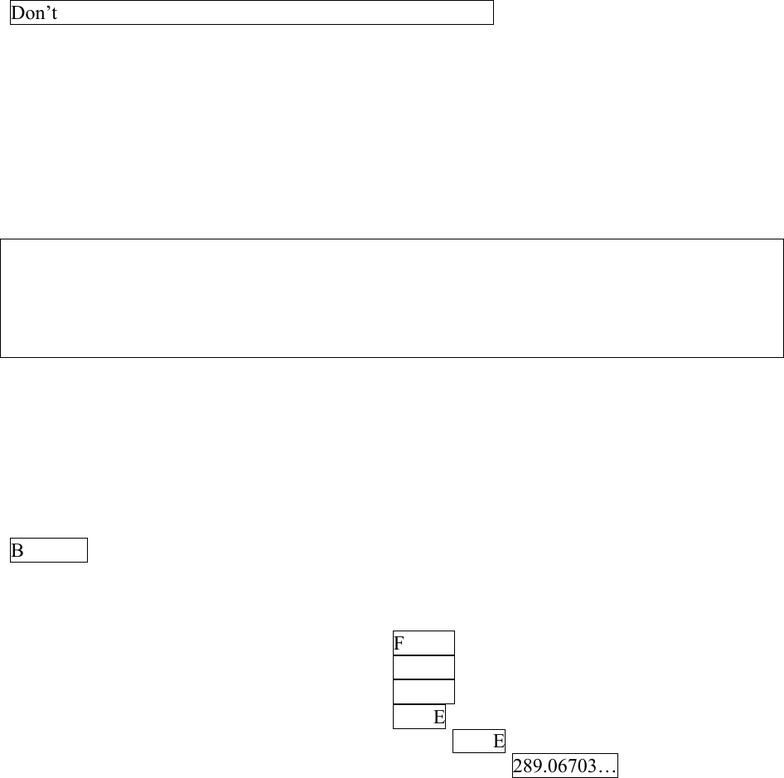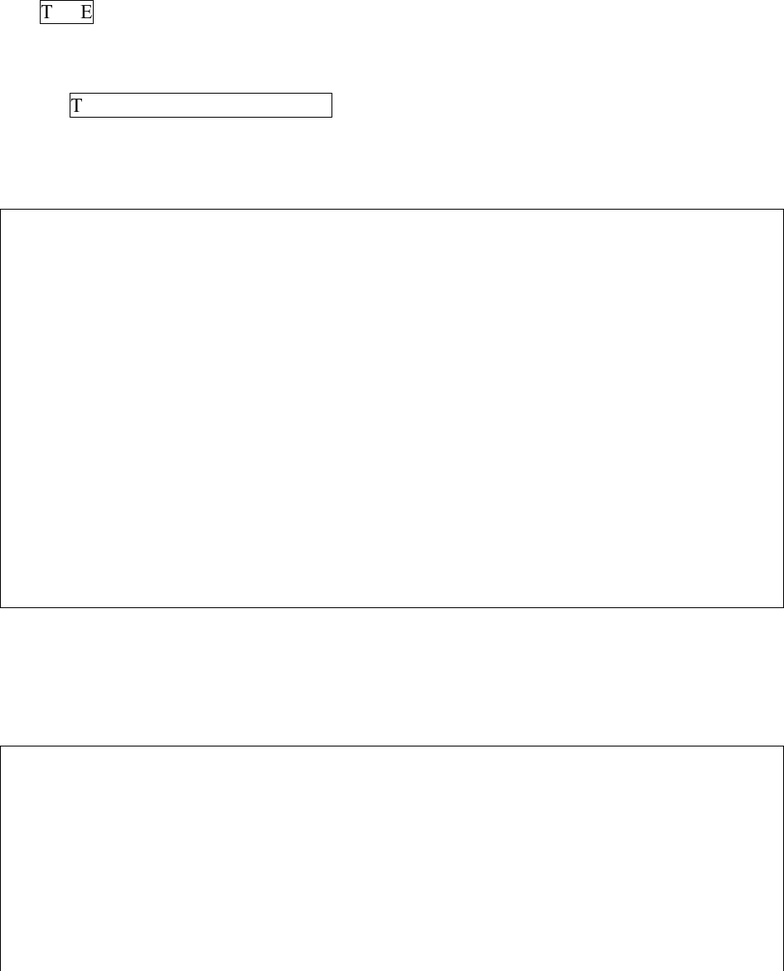Textbook Notes (290,000)
US (110,000)
LSU (20,000)
IE (100)
All (2)
Chapter

# Assignment 02 SolutionsExam

Department
Industrial Engineering
Course Code
IE 2060
Professor
All

This preview shows page 1. to view the full 5 pages of the document.IE2060 Assignment #2 Solutions
CHAPTER 2
60. Find the final value of X
Dim X, N as integer
Dim M as single
M=42.3
N=3
X=X+(M/N)^N + Math.Log(M)/8.3^2 + 12*M
Don’t forget to round off (X is integer) X=3311
62. Find the final value of Test
Dim N As Integer = 14
Dim M As Integer = 45
Dim Test As Boolean
Test = (N > 14) Or (13 > 15) And ( (M mod 2 > 0) _
Or Not (M <> N) ) Or (M*3 > 100)
Test:
= FALSE OR FALSE AND (TRUE OR NOT TRUE) OR TRUE
=FALSE OR (FALSE AND TRUE) OR TRUE ‘AND has higher priority than OR
=FALSE OR FALSE OR TRUE
=TRUE
64. What is the value of B after executing the following statements?
Dim B As Boolean, X As Double=4.3
B = Not B And (X*3/45^3 <1 Or X >3)
B = (NOT FALSE) AND ( (.0001 < 1) OR (4.3 > 3)
B = TRUE AND (TRUE OR TRUE)
B=TRUE
66. Given that X=4.5, Y=3.2, Z=1 and B=False (Boolean), evaluate each of the following Boolean
expressions:
a. (X>3 Or Y<3) And Z>4 Or B FALSE
b. X<=4 Or Z > 1 And Not B FALSE
c. X<=4 Or (Z > 1 And Not B) FALSE
d. X<=4 Or ((Z/0) > 1 And Not B) TRUE
e. X<=4 OrElse ((Z/0) > 1 AndAlso Not B) TRUE
68. B = 13 ^ (-(4 + 6) / 8) + (92 * Math.Pi 145 ^ ( -4 + 6 / 8)) 289.06703…

Unlock to view full version

Only page 1 are available for preview. Some parts have been intentionally blurred.73. W = X And Not Y Or Z And Not Not Z Or 8 <> 2
( TRUE AND (NOT TRUE) ) OR (TRUE AND (NOT (NOT TRUE))) OR (8<>2)
FALSE OR (TRUE AND (NOT FALSE)) OR TRUE
FALSE OR TRUE OR TRUE
TRUE
75. T = “Adding “ & N & “ + “ & M &yields “ & (M+N)
T "Adding 2 + 4.5 yields 6.5"
81. Write a console application that reads in degrees Fahrenheit from the user, converts it to the
equivalent degrees Celsius, and outputs the result to the console window.
Sub Main()
'Variable declarations
Dim S As String
Dim TempF, TempC As Double
'Get temperature in Fahrenheit
'(note: you did not have to check for errors in the previous assignments- you will in later assignments)
Console.Write("Enter temperature in degrees Fahrenheit: ")
Do Until IsNumeric(S)
Console.Write("Value must be numeric. Please re-enter temperature (in degrees F): ")
Loop
TempF = CDbl(S)
'Calculate and write out temperature in Celsius
TempC = (5.0 / 9.0) * (TempF - 32.0)
Console.WriteLine("The temperature in Celsius is " & TempC)
Console.WriteLine("Press [ENTER] to complete program")
End Sub
82. Write a console program to calculate the number of square feet of shingles needed to cover a
gable (upside down V) roof. From the user, read in a) the length of the roof, b) the width of the
roof, and c) the rise (number of feet roof rises vertically for every foot horizontally). To
simplify, assume there are no eaves (overhang).
Sub Main()
'Variable declarations
Dim S As String
Dim Length, Width, Rise, Area As Double
'Get Length, Width, Rise from user; check for errors
Console.WriteLine("Enter the length of the roof ")